# Tamilnadu Board Class 10 Maths Solutions Chapter 2 Numbers and Sequences Ex 2.7

## Tamilnadu State Board Class 10 Maths Solutions Chapter 2 Numbers and Sequences Ex 2.7

Question 1.
Which of the following sequences are in G.P?
(i) 3, 9, 27, 81,…
(ii) 4, 44, 444, 4444,…
(iii) 0.5, 0.05, 0.005,…
(iv) $$\frac { 1 }{ 3 }$$,$$\frac { 1 }{ 6 }$$,$$\frac { 1 }{ 12 }$$………
(v) 1, -5, 25, -125,…
(vi) 120, 60, 30, 18,…
(vii) 16, 4, 1, $$\frac { 1 }{ 4 }$$, ….
Solution:
(i) 3, 9, 27, 81
r = Common ratio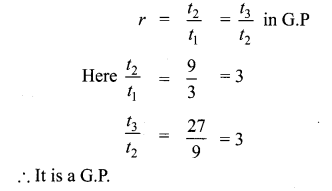(ii) 4, 44, 444, 4444,……(iii) 0.5, 0.05, 0.005,……(iv) $$\frac { 1 }{ 3 }$$, $$\frac { 1 }{ 6 }$$, $$\frac { 1 }{ 12 }$$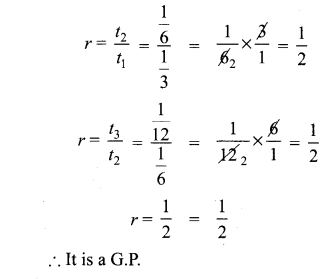(v) 1, – 5, 25, – 125(vi) 120,60,30,18,….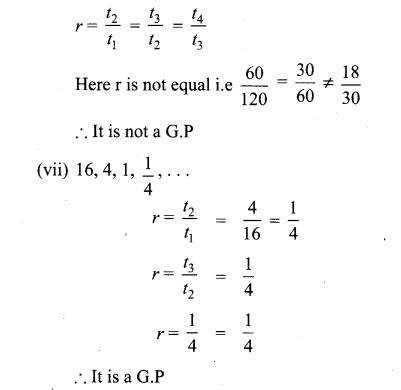Question 2.
Write the first three terms of the G.P. whose first term and the common ratio are given below.
(i) a = 6, r = 3
(ii) a = $$\sqrt { 2 }$$ , r = $$\sqrt { 2 }$$
(iii) a = 1000, r = $$\frac { 2 }{ 5 }$$
Solution:
(i) a = 6, r = 3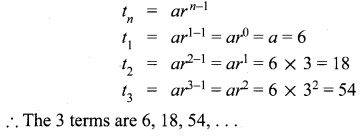(ii) a = $$\sqrt { 2 }$$ , r = $$\sqrt { 2 }$$(iii) a = 1000, r = $$\frac { 2 }{ 5 }$$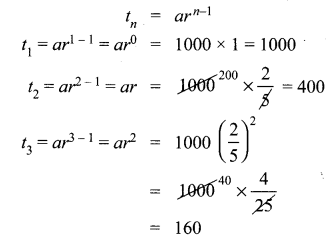The 3 terms are 1000, 400, 160, ……………

Question 3.
In a G.P. 729, 243, 81,… find t7.
Solution:
G.P = 729, 243, 81 ……
t7 = ?Question 4.
Find x so that x + 6, x + 12 and x + 15 are consecutive terms of a Geometric Progression
Solution:
G.P = x + 6, x + 12, x + 15
In G.P r = $$\frac{t_{2}}{t_{1}}=\frac{t_{3}}{t_{2}}$$
$$\frac{x+12}{x+6}=\frac{x+15}{x+12}$$
(x + 12)2 = (x + 6) (x + 5)
x2 + 24x + 144 = x2 + 6x + 15x + 90
24x – 21x = 90 – 144
3x = -54
x = $$\frac { -54 }{ 3 }$$ = -18
x = -18

Question 5.
Find the number of terms in the following G.P.
(i),4,8,16,…,8192
(ii) $$\frac { 1 }{ 3 }$$,$$\frac { 1 }{ 9 }$$,$$\frac { 1 }{ 27 }$$,……$$\frac { 1 }{ 2187 }$$
Solution:
(i) 4, 8, 16, …… 8192Question 6.
In a G.P. the 9th term is 32805 and 6th term is 1215. Find the 12th term.
Solution:
In a G.P
tn = arn-1
t9 = 32805
t6 = 1215
t12 = ?
Let
t9 = ar8 = 32805 ….(1)
t6 = ar5 = 1215 ….(2)Question 7.
Find the 10th term of a G.P. whose 8th term is 768 and the common ratio is 2.
Solution:
t8 = 768 = ar7
r = 2
t10 = ar9 = ar7 × r × r
= 768 × 2 × 2 = 3072

Question 8.
If a, b, c are in A.P. then show that 3a,3b,3c are in G.P.
Solution:
If a, b, c are in A.P
t2 – t1 = t3 – t2
b – a = c – b
2b = c + a
To prove that 3a, 3b, 3c are in G.P
⇒ 32b = 3c+a + a [Raising the power both sides]
⇒ 3b.3b = 3c.3a
$$\Rightarrow \frac{3^{b}}{3^{a}}=\frac{3^{c}}{3^{b}}$$
$$\Rightarrow \frac{t_{2}}{t_{1}}=\frac{t_{3}}{t_{1}}$$
⇒ Common ratio is same for 3a,3b,3c
⇒ 3a,3b,3c forms a G.P
∴ Hence it is proved .

Question 9.
In a G.P. the product of three consecutive terms is 27 and the sum of the product of two terms taken at a time is $$\frac { 57 }{ 2 }$$. Find the three terms.
Solution:
Let the three consecutive terms in a G.P are $$\frac { a }{ r }$$, a, ar.
Their Product = $$\frac { a }{ r }$$ × a × ar = 27
a3 = 27 = 33
a = 3
Sum of the product of terms taken two at a time is $$\frac { 57 }{ 2 }$$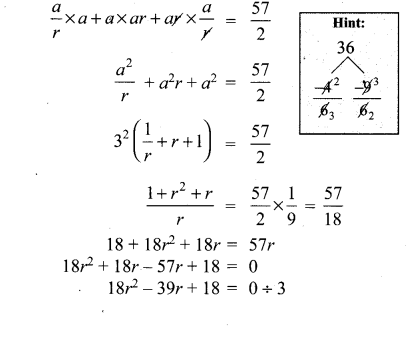Question 10.
A man joined a company as Assistant Manager. The company gave him a starting salary of ₹60,000 and agreed to increase his salary 5% annually. What will be his salary after 5 years?
Solution:
Starting salary = ₹ 60,000
Increase per year = 5%
∴ At the end of 1 year the increase
= 60,0,00 × $$\frac { 5 }{ 100 }$$
₹ 3000
∴ At the end of first year his salary
= ₹ 60,000 + 3000
I year salary = ₹ 63,000
II Year increase = 63000 × $$\frac { 5 }{ 100 }$$
At the end of II year, salary
= 63000 + 3150
= ₹ 66150
III Year increase = 66150 × $$\frac { 5 }{ { 100 }_{ 2 } }$$
= 3307.50
At the end of III year, salary = 66150 + 3307.50
= ₹ 69457.50
IV year increase = 69457.50 × $$\frac { 5 }{ 100 }$$
= ₹ 3472.87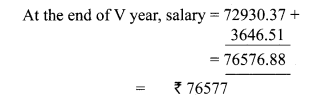Question 11.
Sivamani is attending an interview for a job and the company gave two offers to him. Offer A: ₹ 20,000 to start with followed by a guaranteed annual increase of 3% for the first 5 years.
Offer B: ₹ 22,000 to start with followed by a guaranteed annual increase of 3% for the first 5 years.
What is his salary in the 4th year with respect to the offers A and B?
Solution:
Offer A
Starting salary ₹ 20,000
Annual increase 6%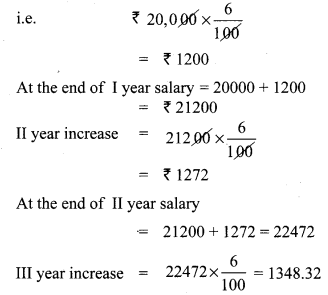At the end of
III year ,salary = 22472 + 1348 = 23820
∴ IV year salary = ₹ 23820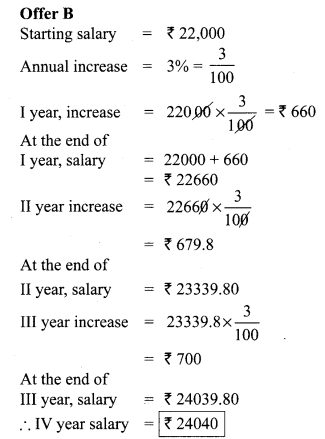Salary as per Option A = ₹ 23820
Salary as per Option B = ₹ 24040
∴ Option B is better.

Question 12.
If a, b, c are three consecutive terms of an A.P. and x, y, z are three consecutive terms of a G.P. then prove that xb-c × yc-a × za-b = 1.
Solution:
a, b, c are three consecutive terms of an AP.
∴ Let a, b, c be a, a + d, a + 2d respectively …(1)
x, y, z are three consecutive terms of a GP.
∴ Assume x, y, z as x, x.r, x.r2 respectively …(2)